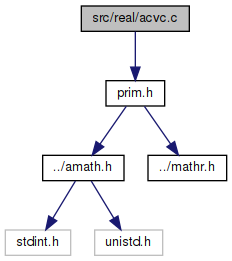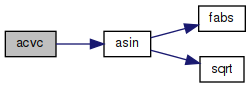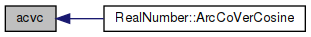amath  1.8.5 Simple command line calculator
acvc.c File Reference

Inverse versed cosine. More...

`#include "prim.h"`
Include dependency graph for acvc.c:Go to the source code of this file.

## Functions

double acvc (double x)
Inverse versed cosine. More...

## Detailed Description

Inverse versed cosine.

Definition in file acvc.c.

## ◆ acvc()

 double acvc ( double x )

Inverse versed cosine.

```Method
acvc(x) = asin(1+x)
```

Definition at line 45 of file acvc.c.

References asin().

Referenced by RealNumber::ArcCoVerCosine().

46 {
47  return asin(1.0 + x);
48 }
double asin(double x)
Inverse sine function.
Definition: asin.c:100
Here is the call graph for this function:Here is the caller graph for this function: